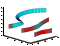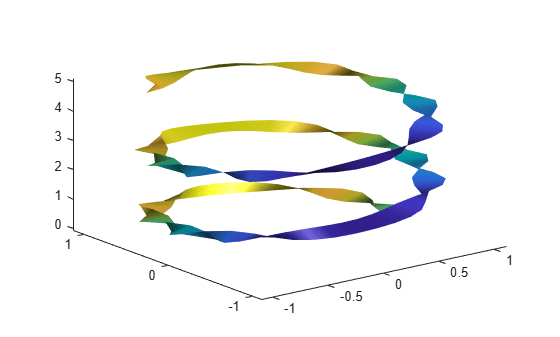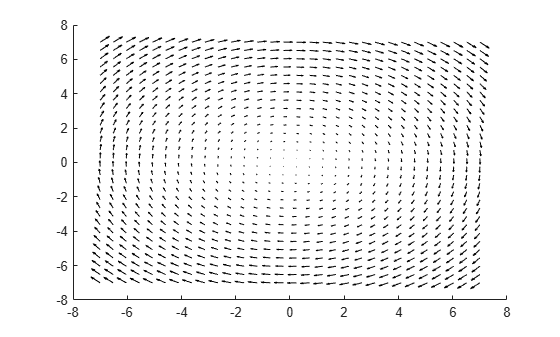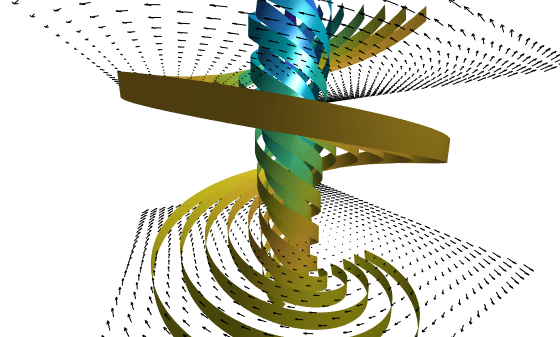# streamribbon

3-D stream ribbon plot from vector volume data## Syntax

```streamribbon(X,Y,Z,U,V,W,startx,starty,startz) streamribbon(U,V,W,startx,starty,startz) streamribbon(vertices,X,Y,Z,cav,speed) streamribbon(vertices,cav,speed) streamribbon(vertices,twistangle) streamribbon(...,width) streamribbon(axes_handle,...) h = streamribbon(...) ```

## Description

`streamribbon(X,Y,Z,U,V,W,startx,starty,startz)` draws stream ribbons from vector volume data `U`, `V`, `W`.

The arrays `X`, `Y`, and `Z`, which define the coordinates for `U`, `V`, and `W`, must be monotonic, but do not need to be uniformly spaced. `X`, `Y`, and `Z` must have the same number of elements, as if produced by `meshgrid`.

`startx`, `starty`, and `startz` define the starting positions of the stream ribbons at the center of the ribbons.

The twist of the ribbons is proportional to the curl of the vector field. The width of the ribbons is calculated automatically.

`streamribbon(U,V,W,startx,starty,startz)` assumes `X`, `Y`, and `Z` are determined by the expression

```[X,Y,Z] = meshgrid(1:n,1:m,1:p) ```

where `[m,n,p] = size(U)`.

`streamribbon(vertices,X,Y,Z,cav,speed)` assumes precomputed streamline vertices, curl angular velocity, and flow speed. `vertices` is a cell array of streamline vertices (as produced by `stream3`). `X`, `Y`, `Z`, `cav`, and `speed` are 3-D arrays.

`streamribbon(vertices,cav,speed)` assumes `X`, `Y`, and `Z` are determined by the expression

```[X,Y,Z] = meshgrid(1:n,1:m,1:p) ```

where `[m,n,p] = size(cav)`.

`streamribbon(vertices,twistangle)` uses the cell array of vectors `twistangle` for the twist of the ribbons (in radians). The size of each corresponding element of `vertices` and `twistangle` must be equal.

`streamribbon(...,width)` sets the width of the ribbons to `width`.

`streamribbon(axes_handle,...)` plots into the axes object with the handle `axes_handle` instead of into the current axes object (`gca`).

`h = streamribbon(...)` returns a vector of handles (one per start point) to `surface` objects.

## Examples

collapse all

Use stream ribbons to indicate the flow in a data set.

```load wind [sx,sy,sz] = meshgrid(80,20:10:50,0:5:15); streamribbon(x,y,z,u,v,w,sx,sy,sz); axis tight shading interp view(3); camlight lighting gouraud```Use precalculated vertex data, curl average velocity, and speed to indicate flow.

```load wind [sx,sy,sz] = meshgrid(80,20:10:50,0:5:15); verts = stream3(x,y,z,u,v,w,sx,sy,sz); cav = curl(x,y,z,u,v,w); spd = sqrt(u.^2 + v.^2 + w.^2).*.1; streamribbon(verts,x,y,z,cav,spd); axis tight shading interp view(3); camlight; lighting gouraud```Using precalculated data enables you to use values other than those calculated from the single data source. In this case, the speed is reduced by a factor of 10 compared to the previous example.

Specify a twist angle for the stream ribbon

```t = 0:.15:15; verts = {[cos(t)' sin(t)' (t/3)']}; twistangle = {cos(t)'}; streamribbon(verts,twistangle); axis tight shading interp view(3) camlight lighting gouraud```Create 3-D arrays and a cone plot.

```xmin = -7; xmax = 7; ymin = -7; ymax = 7; zmin = -7; zmax = 7; x = linspace(xmin,xmax,30); y = linspace(ymin,ymax,20); z = linspace(zmin,zmax,20); [x,y,z] = meshgrid(x,y,z); u = y; v = -x; w = 0*x+1; [cx,cy,cz] = meshgrid(linspace(xmin,xmax,30),... linspace(ymin,ymax,30),[-3 4]); h = coneplot(x,y,z,u,v,w,cx,cy,cz,'quiver'); set(h,'Color','k');```Plot two sets of stream ribbons. Then define the view and lighting.

```[sx,sy,sz] = meshgrid([-1 0 1],[-1 0 1],-6); streamribbon(x,y,z,u,v,w,sx,sy,sz); [sx,sy,sz] = meshgrid([1:6],,-6); streamribbon(x,y,z,u,v,w,sx,sy,sz); shading interp view(-30,10) axis off tight camproj perspective camva(66) camlookat camdolly(0,0,.5,'fixtarget') camlight```